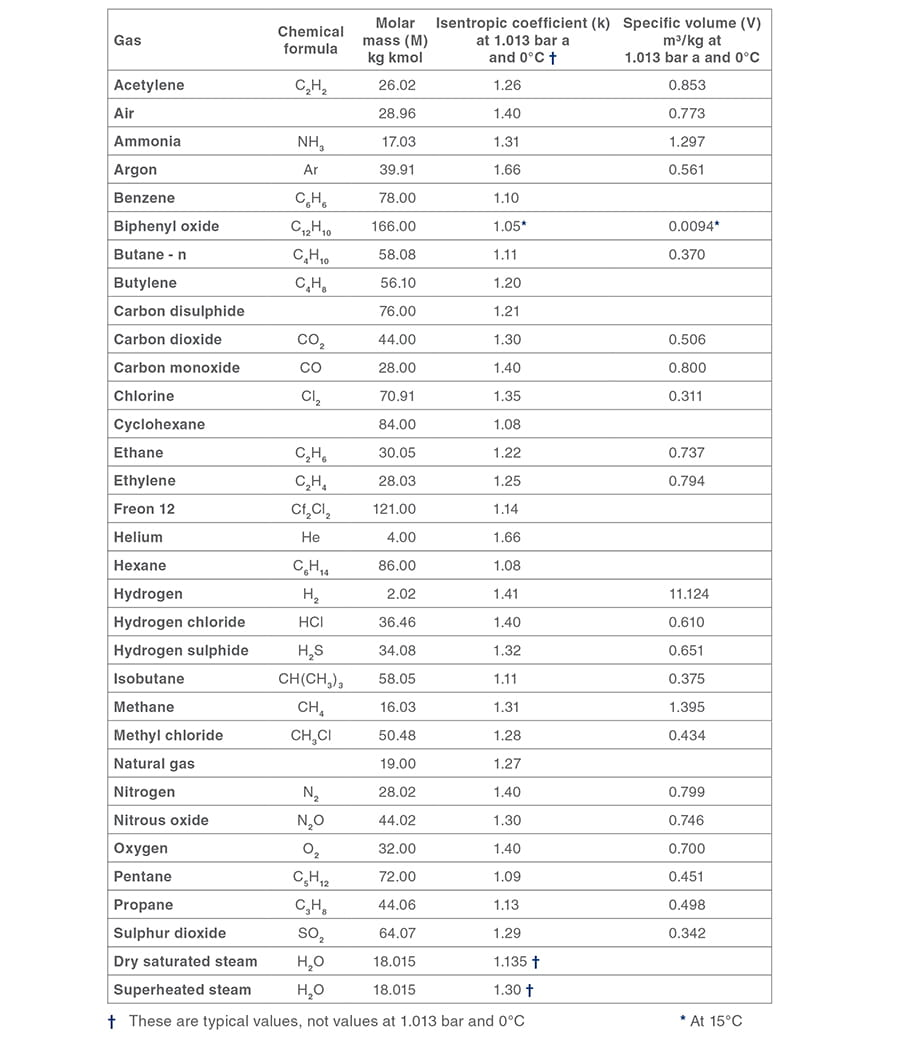# Safety Valves

## Safety Valve Sizing

An in-depth study of the sizing process for a range of applications, including sizing equations for AD Merkblatt, DIN , TRD, ASME, API, BS6759 and others. Covers more complex issues such as two-phase flow and superheat.

#### Safety Valve Sizing Introduction

A safety valve must always be sized and able to vent any source of steam so that the pressure within the protected apparatus cannot exceed the maximum allowable accumulated pressure (MAAP). This not only means that the valve has to be positioned correctly, but that it is also correctly set. The safety valve must then also be sized correctly, enabling it to pass the required amount of steam at the required pressure under all possible fault conditions.

Once the type of safety valve has been established, along with its set pressure and its position in the system, it is necessary to calculate the required discharge capacity of the valve. Once this is known, the required orifice area and nominal size can be determined using the manufacturer’s specifications.

In order to establish the maximum capacity required, the potential flow through all the relevant branches, upstream of the valve, need to be considered.

In applications where there is more than one possible flow path, the sizing of the safety valve becomes more complicated, as there may be a number of alternative methods of determining its size. Where more than one potential flow path exists, the following alternatives should be considered:

• The safety valve can be sized on the maximum flow experienced in the flow path with the greatest amount of flow.
• The safety valve can be sized to discharge the flow from the combined flow paths.

This choice is determined by the risk of two or more devices failing simultaneously. If there is the slightest chance that this may occur, the valve must be sized to allow the combined flows of the failed devices to be discharged. However, where the risk is negligible, cost advantages may dictate that the valve should only be sized on the highest fault flow. The choice of method ultimately lies with the company responsible for insuring the plant.

For example, consider the pressure vessel and automatic pump-trap (APT) system as shown in Figure 9.4.1. The unlikely situation is that both the APT and pressure reducing valve (PRV ‘A’) could fail simultaneously. The discharge capacity of safety valve ‘A’ would either be the fault load of the largest PRV, or alternatively, the combined fault load of both the APT and PRV ‘A’.

This document recommends that where multiple flow paths exist, any relevant safety valve should, at all times, be sized on the possibility that relevant upstream pressure control valves may fail simultaneously.Finding the fault flow

In order to determine the fault flow through a PRV or indeed any valve or orifice, the following need to be considered:

• The potential fault pressure - this should be taken as the set pressure of the appropriate upstream safety valve
• The relieving pressure of the safety valve being sized
• The full open capacity (KVS) of the upstream control valve, see Equation 3.21.2

Example 9.4.1

Consider the PRV arrangement in Figure 9.4.2.The supply pressure of this system (Figure 9.4.2) is limited by an upstream safety valve with a set pressure of 11.6 bar g. The fault flow through the PRV can be determined using the steam mass flow equation (Equation 3.21.2):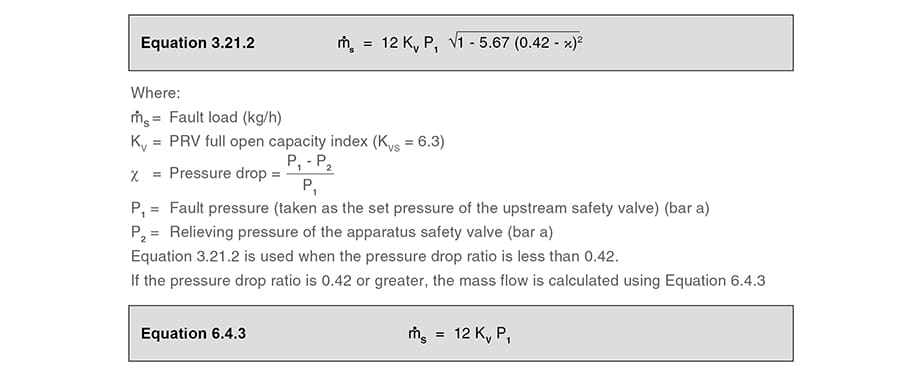In this example: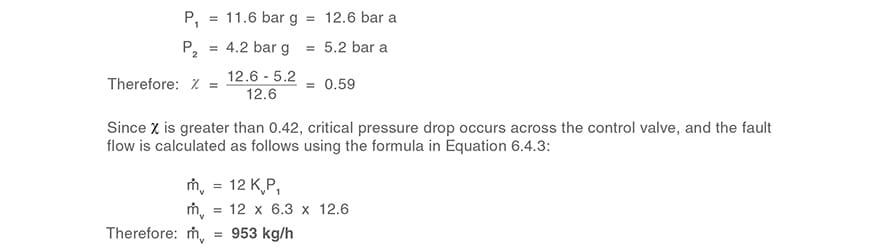Consequently, the safety valve would be sized to pass at least 953 kg/h when set at 4 bar g.

Once the fault load has been determined, it is usually sufficient to size the safety valve using the manufacturer’s capacity charts. A typical example of a capacity chart is shown in Figure 9.4.3. By knowing the required set pressure and discharge capacity, it is possible to select a suitable nominal size. In this example, the set pressure is 4 bar g and the fault flow is 953 kg/h. A DN32/50 safety valve is required with a capacity of 1 284 kg/h.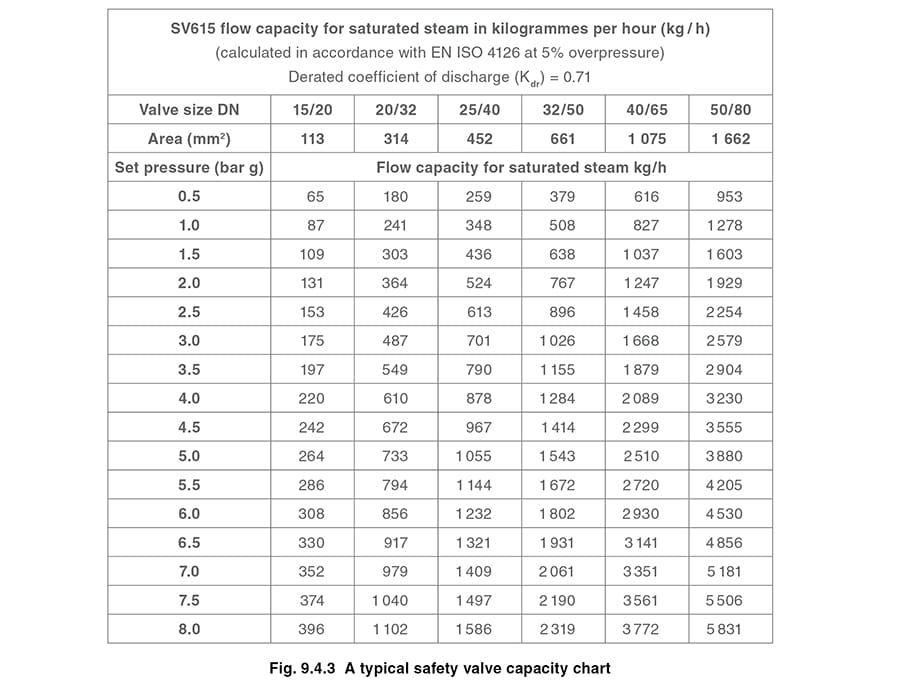Where sizing charts are not available or do not cater for particular fluids or conditions, such as backpressure, high viscosity or two-phase flow, it may be necessary to calculate the minimum required orifice area. Methods for doing this are outlined in the appropriate governing standards, such as:

• AD-Merkblatt A2, DIN 3320, TRD 421
• ASME/API RP 520
•  EN ISO 4126

The methods outlined in these standards are based on the coefficient of discharge, which is the ratio of the measured capacity to the theoretical capacity of a nozzle with an equivalent flow area.Coefficient of discharge

Coefficients of discharge are specific to any particular safety valve range and will be approved by the manufacturer. If the valve is independently approved, it is given a ‘certified coefficient of discharge’.

This figure is often derated by further multiplying it by a safety factor 0.9, to give a derated coefficient of discharge. Derated coefficient of discharge is termed Kdr = Kd x 0.9

When using standard methods of calculating the required orifice area, the following points may need to be considered:

Critical and sub-critical flow - the flow of gas or vapour through an orifice, such as the flow area of a safety valve, increases as the downstream pressure is decreased. This holds true until the critical pressure is reached, and critical flow is achieved. At this point, any further decrease in the downstream pressure will not result in any further increase in flow.

A relationship (called the critical pressure ratio) exists between the critical pressure and the actual relieving pressure, and, for gases flowing through safety valves, is shown by Equation 9.4.2.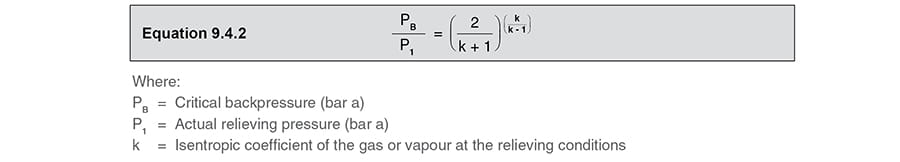For gases, with similar properties to an ideal gas, ‘k’ is the ratio of specific heat of constant pressure (cp) to constant volume (cv), i.e. cp : cv. ‘k’ is always greater than unity, and typically between 1 and 1.4 (see Table 9.4.8).

For steam, although ‘k’ is an isentropic coefficient, it is not actually the ratio of cp : c. As an approximation for saturated steam, ‘k’ can be taken as 1.135, and superheated steam, as 1.3. As a guide, for saturated steam, critical pressure is taken as 58% of accumulated inlet pressure in absolute terms.

• Overpressure - Before sizing, the design overpressure of the valve must be established. It is not permitted to calculate the capacity of the valve at a lower overpressure than that at which the coefficient of discharge was established. It is however, permitted to use a higher overpressure (see Table 9.2.1, Module 9.2, for typical overpressure values). For DIN type full lift (Vollhub) valves, the design lift must be achieved at 5% overpressure, but for sizing purposes, an overpressure value of 10% may be used.

For liquid applications, the overpressure is 10% according to AD-Merkblatt A2, DIN 3320, TRD 421 and ASME, but for non-certified ASME valves, it is quite common for a figure of 25% to be used.

• Backpressure - The sizing calculations in the AD-Merkblatt A2, DIN 3320 and TRD 421 standards account for backpressure in the outflow function,(Ψ), which includes a backpressure correction.

The ASME/API RP 520 and EN ISO 4126 standards, however, require an additional backpressure correction factor to be determined and then incorporated in the relevant equation.

• Two-phase flow - When sizing safety valves for boiling liquids (e.g. hot water) consideration must be given to vaporisation (flashing) during discharge. It is assumed that the medium is in liquid state when the safety valve is closed and that, when the safety valve opens, part of the liquid vaporises due to the drop in pressure through the safety valve. The resulting flow is referred to as two-phase flow.

The required flow area has to be calculated for the liquid and vapour components of the discharged fluid. The sum of these two areas is then used to select the appropriate orifice size from the chosen valve range. (see Example 9.4.3)

Many standards do not actually specify sizing formula for two-phase flow and recommend that the manufacturer be contacted directly for advice in these instances.

#### Sizing equations for safety valves designed to the following standards

The following methods are used to calculate the minimum required orifice area for a safety valve, as mentioned in the most commonly used national standards.

##### Standard - AD-Merkblatt A2, DIN 3320, TRD 421

Use Equation 9.4.3 to calculate the minimum required orifice area for a safety valve used on steam applications:Use Equation 9.4.4 to calculate the minimum required orifice area for a safety valve used on air and gas applications:Use Equation 9.4.5 to calculate the minimum required orifice area for a safety valve used on liquid applications: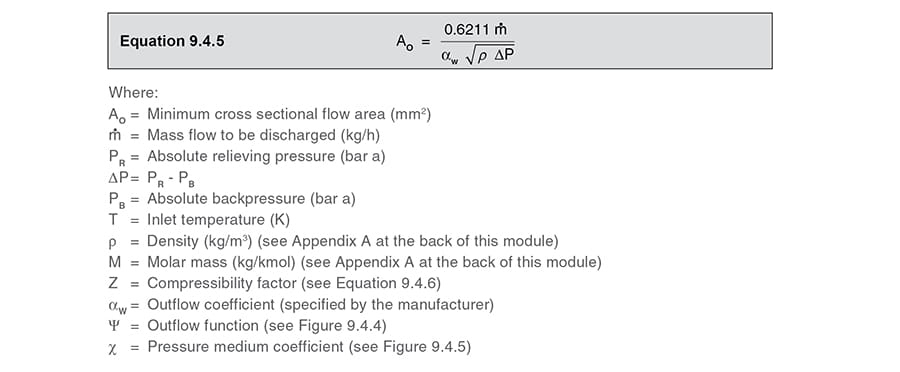The outflow function (Ψ) for air and gas applications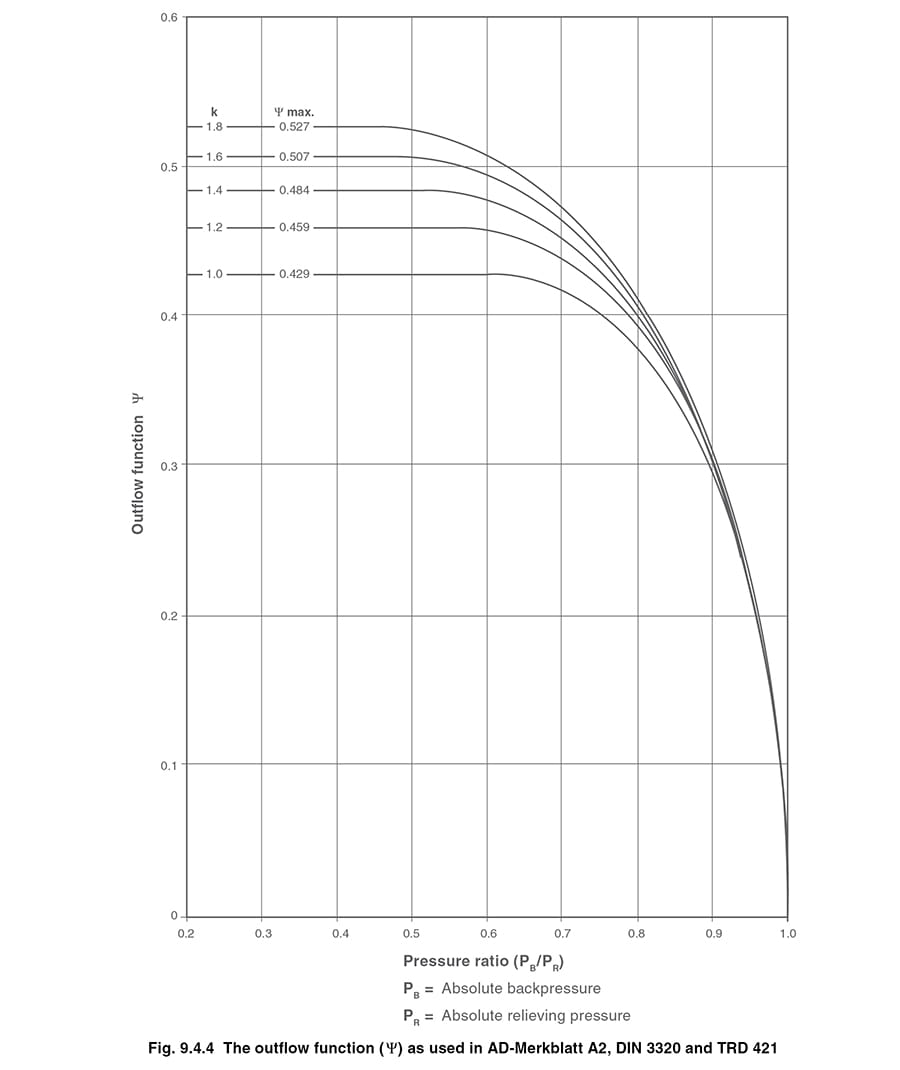#### Pressure medium coefficient for steam applications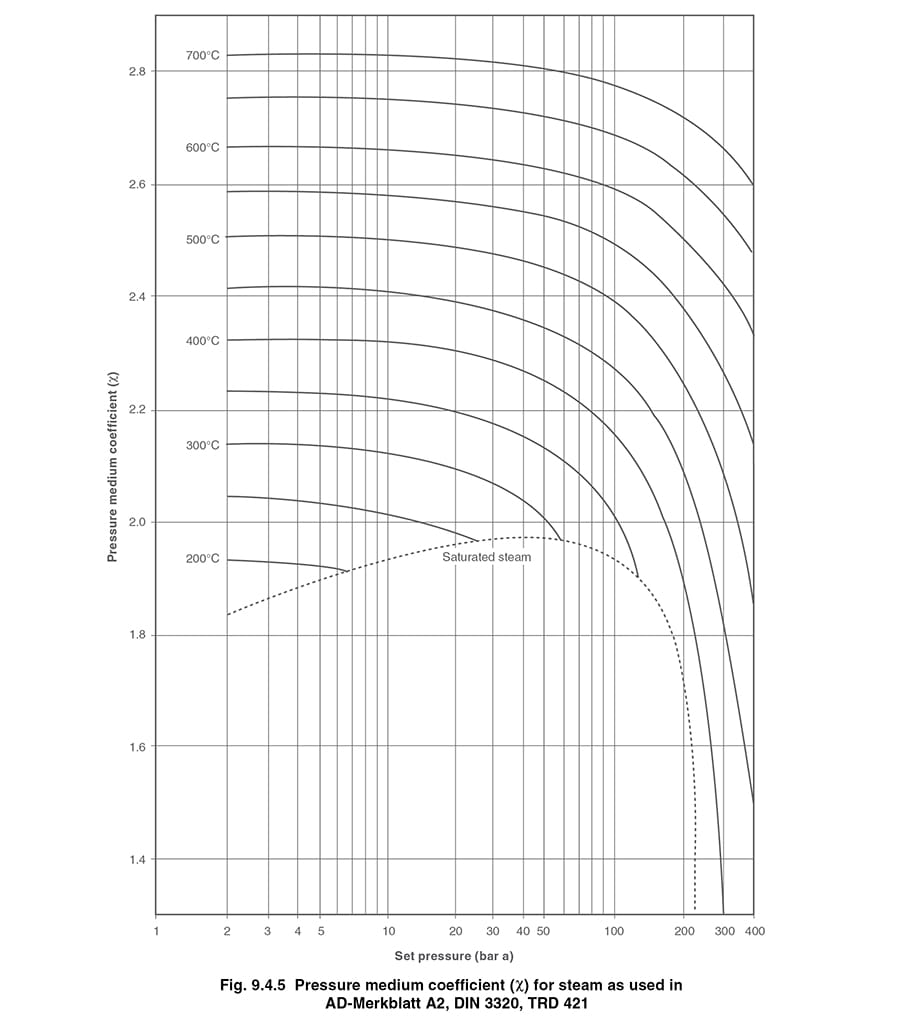##### Compressibility factor (Z)

For gases, the compressibility factor, Z, also needs to be determined. This factor accounts for the deviation of the actual gas from the characteristics of an ideal gas. It is often recommended that Z = 1 is used where insufficient data is available. Z can be calculated by using the formula in Equation 9.4.6: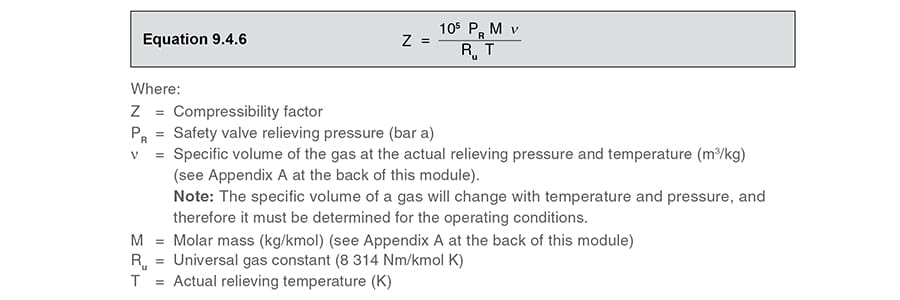Example 9.4.2

Determine the minimum required safety valve orifice area under the following conditions: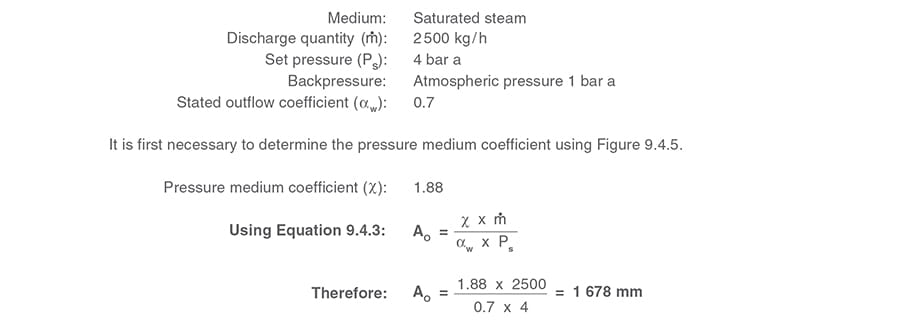Consequently, the chosen safety valve would need an orifice area of at least 1 678 mm2.

Two-phase flow

In order to determine the minimum orifice area for a two-phase flow system (e.g. hot water), it is first necessary to establish what proportion of the discharge will be vapour (n). This is done using the Equation 9.4.7: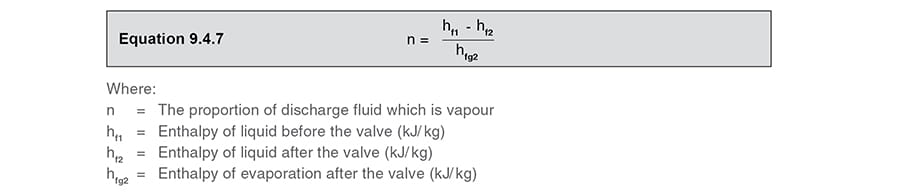For hot water, the enthalpy values can be obtained from steam tables.

In order to determine the proportion of flow, which is vapour, the discharge capacity is multiplied by n. The remainder of the flow will therefore be in the liquid state.

The area sizing calculation from Equations 9.4.3, 9.4.4 and 9.4.5 can then be used to calculate the required area to discharge the vapour portion and then the liquid portion. The sum of these areas is then used to establish the minimum required orifice area.

Example 9.4.3

Consider hot water under the following conditions: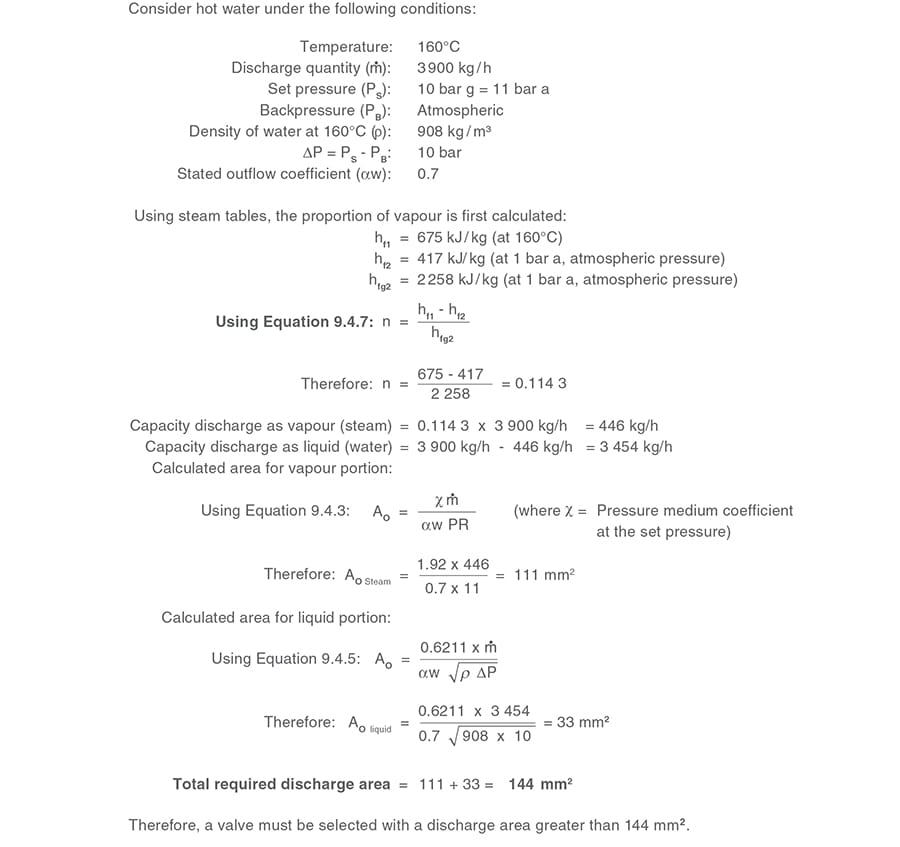##### Standard - ASME /API RP 520

The following formulae are used for calculating the minimum required orifice area for a safety valve according to ASME standards and the API RP 520 guidelines.

Use Equation 9.4.8 to calculate the minimum required orifice area for a safety valve used on steam applications:Use Equation 9.4.9 to calculate the minimum required orifice area for a safety valve ed on air and gas applications:Use Equation 9.4.10 to calculate the minimum required orifice area for a safety valve used on liquid applications: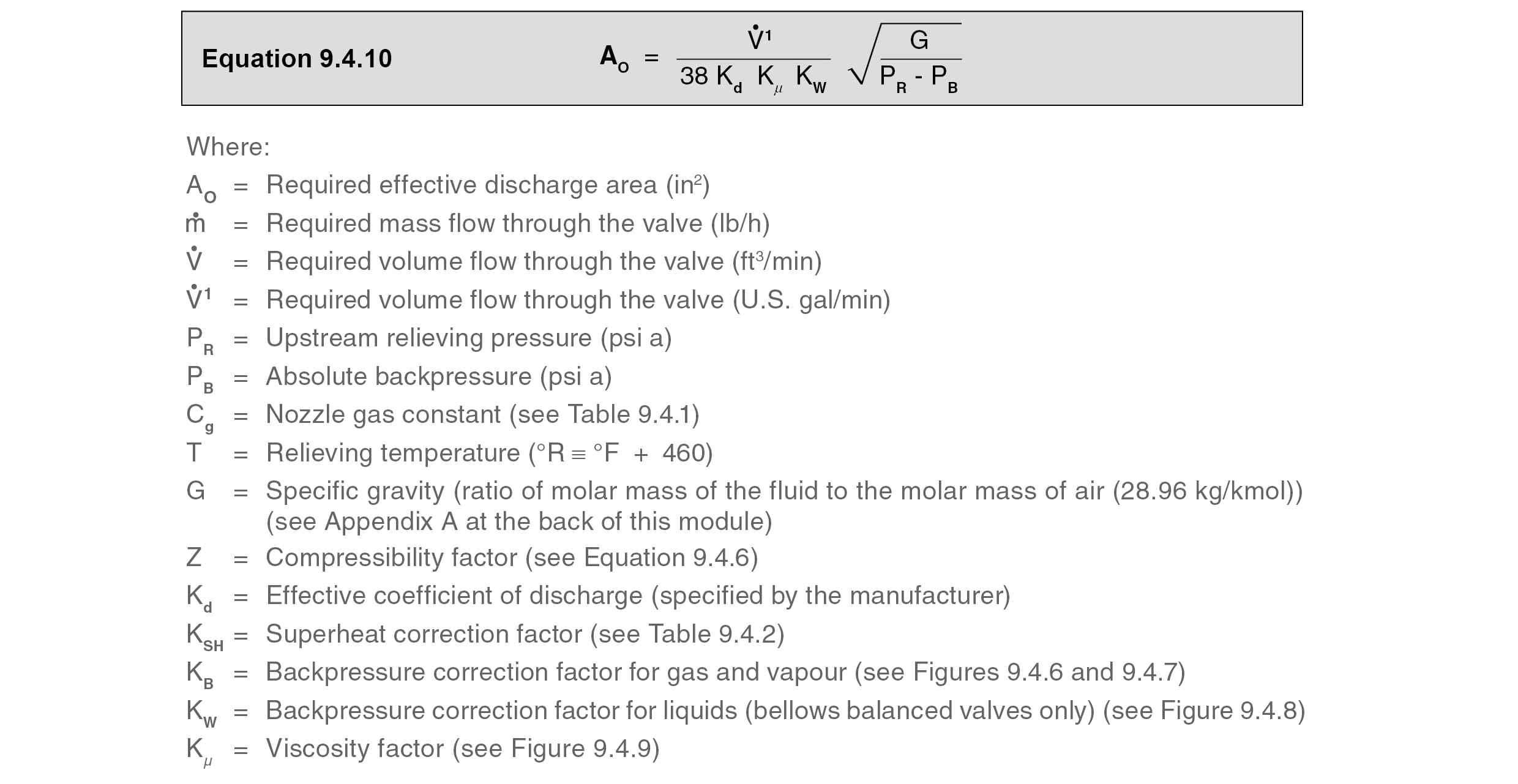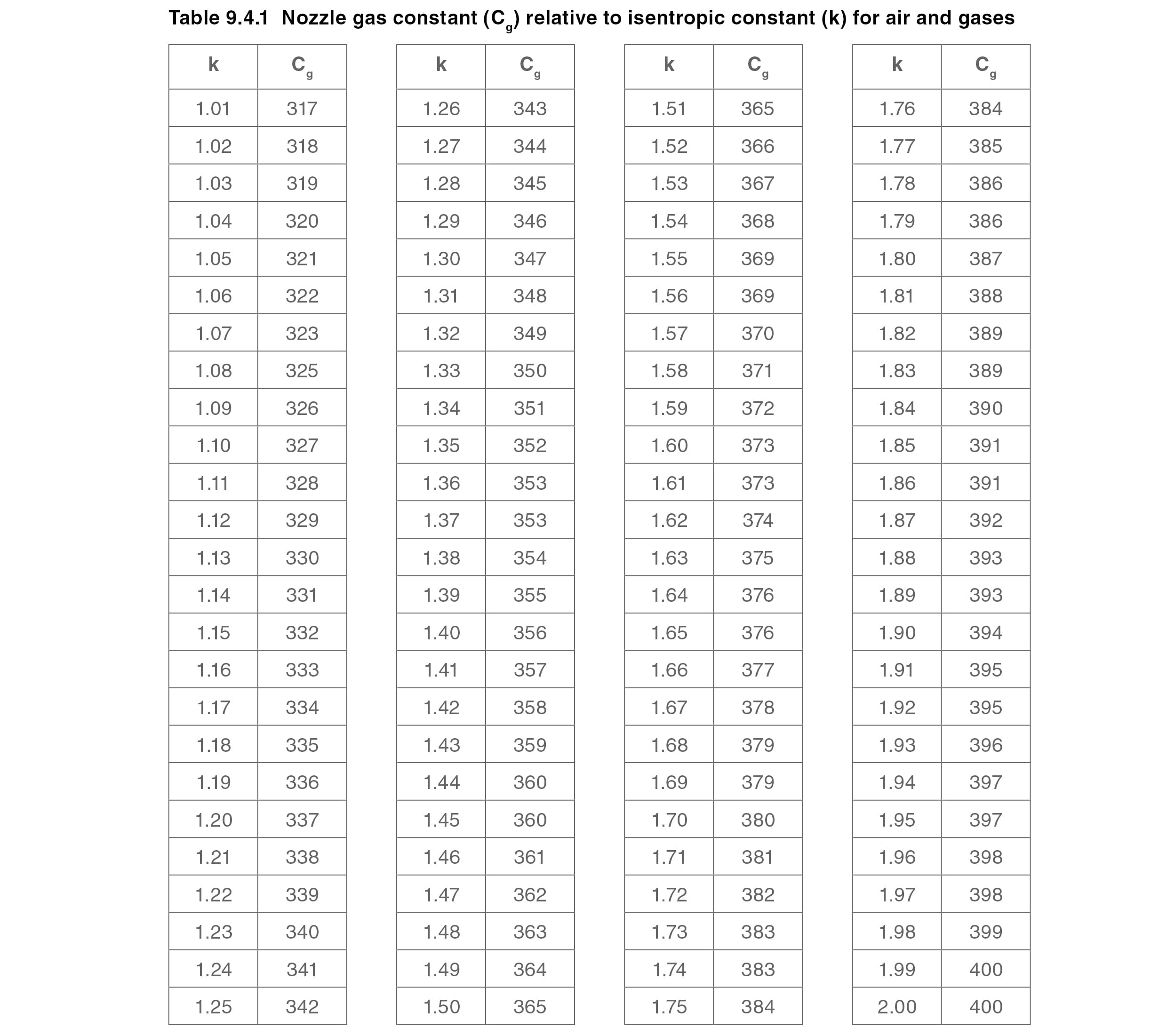The nozzle gas constant Cg is calculated using Equation 9.4.11, for air and gas applications and applied to Equation 9.4.9.##### Superheat correction factors for ASME/API RP 520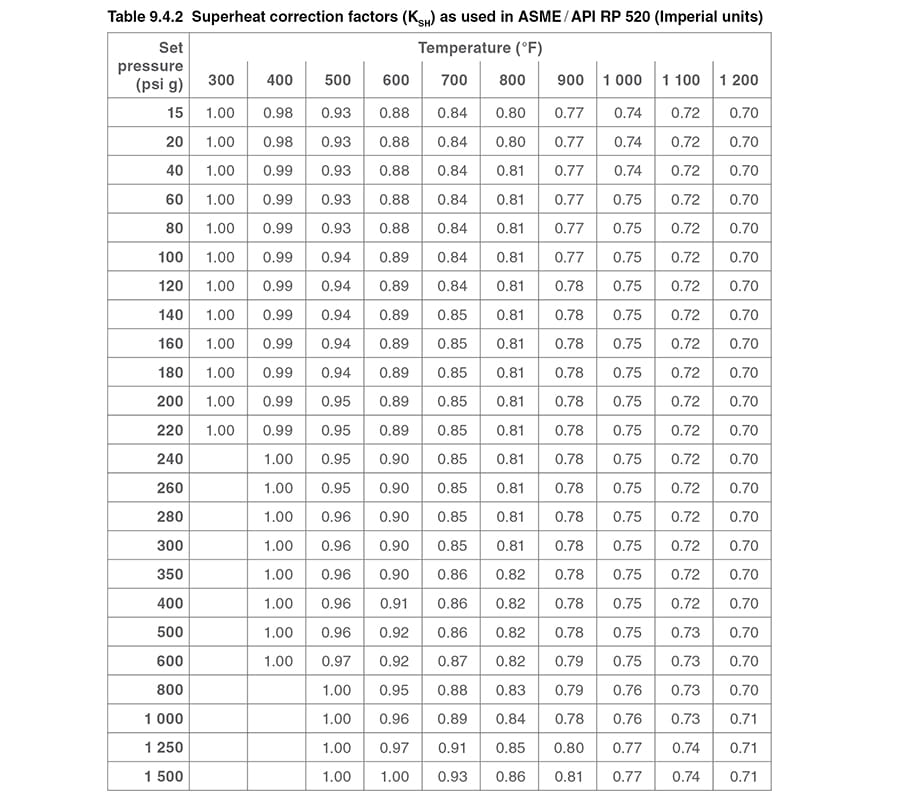##### Gas and vapour constant backpressure correction factor for ASME/API 520

The backpressure correction factor (KB) is the ratio of the capacity with backpressure, C1, to the capacity when discharging to atmosphere, C2, see Equation 9.4.12.The value of KB can be established using the curves shown in Figure 9.4.6 to Figure 9.4.8. These are applicable to set pressures of 50 psi g and above. For a given set pressure, these values are limited to a backpressure less than the critical pressure, namely, critical flow conditions.

For sub-critical flow and backpressures below 50 psi g, the manufacturer should be consulted for values of KB.

##### Balanced bellows valves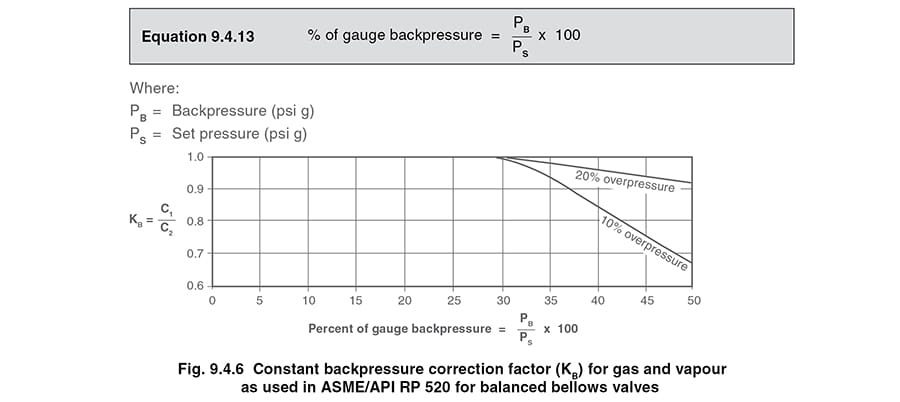##### Conventional valves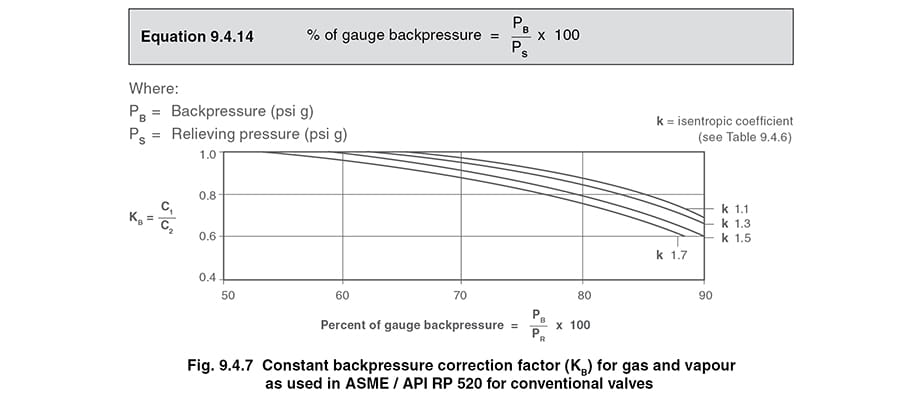##### Balanced bellows valves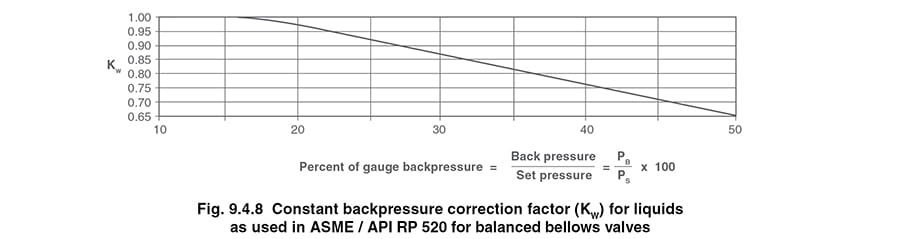##### Viscosity correction factor for ASME/API RP 520 and EN ISO 4126

This is used to make allowances for high viscosity fluids. In order to account for this, the valve size must first be established, assuming the fluid is non-viscous. Once the size has been selected, the Reynolds number for the valve is calculated and used to establish the correction factor from Figure 9.4.9.

The valve size should then be checked to ensure that the original size chosen would accommodate the flow after the viscous correction factor has been applied. If not this process should be repeated with the next largest valve size.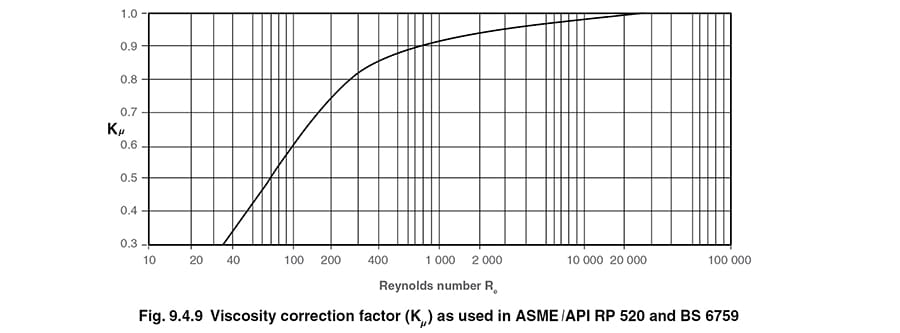The Reynolds number can be calculated using Equations 9.4.15 and 9.4.16: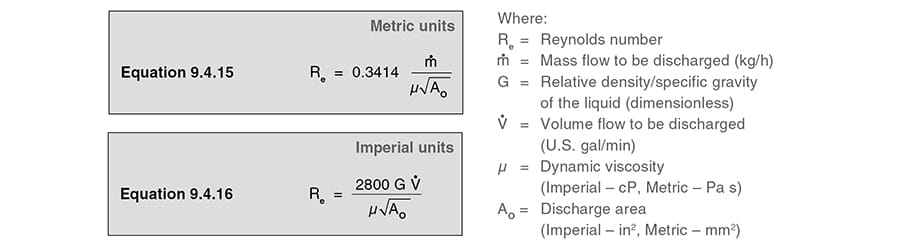##### Standard - EN ISO 4126: 2004

Use Equation 9.4.17 to calculate the minimum required orifice area for a safety valve used on dry saturated steam, superheated steam, and air and gas applications at critical flow:Use Equation 9.4.18 to calculate the minimum required orifice area for a safety valve used on wet steam applications at critical flow; Note: wet steam must have a dryness fraction greater than 0.9:Use Equation 9.4.19 to calculate the minimum required orifice area for a safety valve used on air and gas applications at sub-critical flow:Use Equation 9.4.20 to calculate the minimum required orifice area for a safety valve used on liquid applications: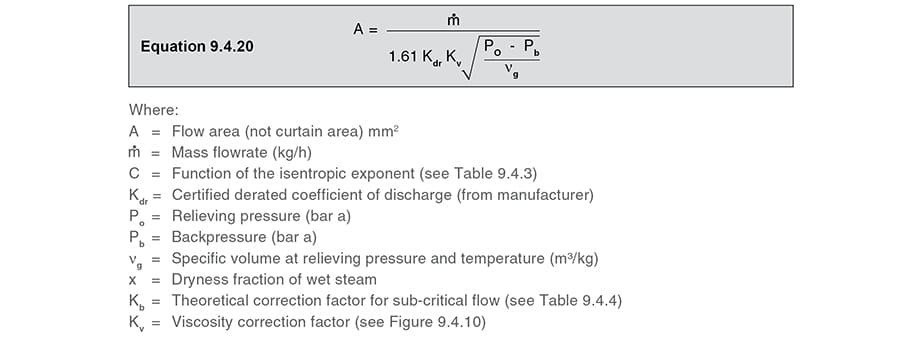Table 9.4.3

Value of C as a function of ‘k’ for steam, air and gas applications to the EN ISO 4126 standard.

‘k’ values are incorporated into the ISO 4126 standard: (Part 7).

Alternatively, ‘k’ values can be obtained from the Spirax Sarco website steam tables.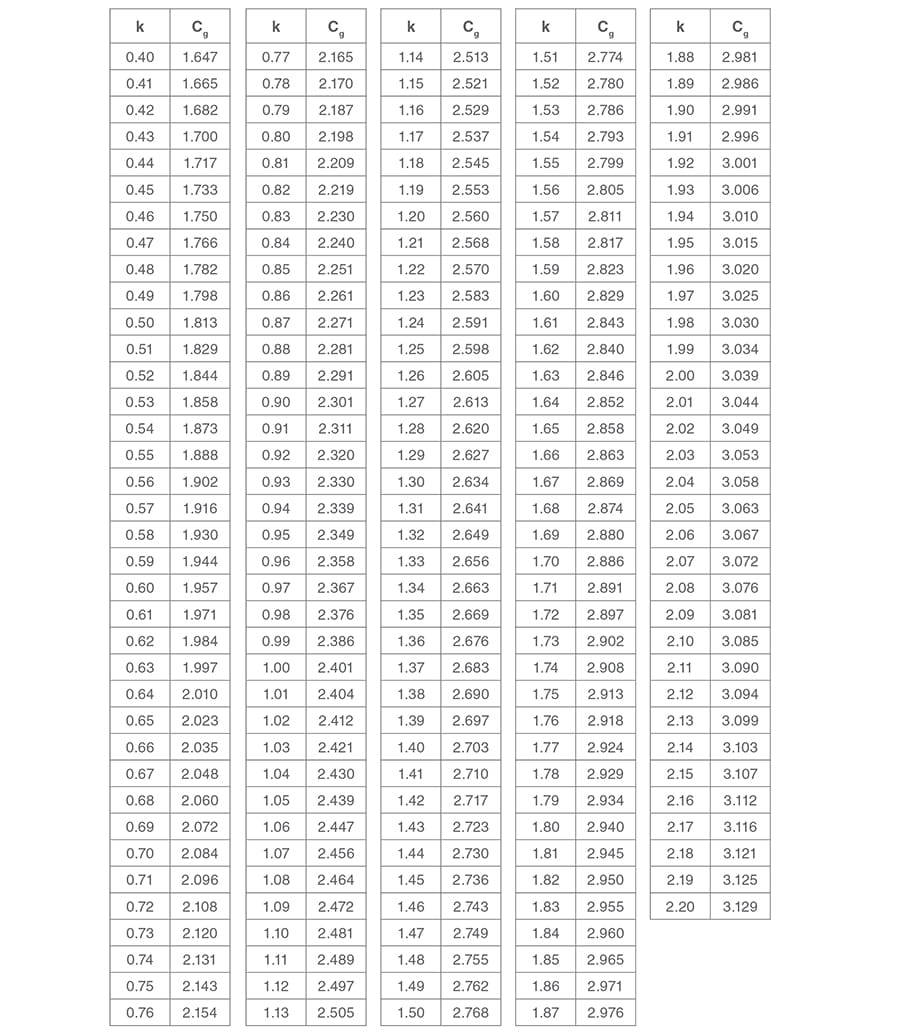Table 9.4.4

Capacity correction factors for backpressure to the EN ISO 4126 standard for steam, air and gas applications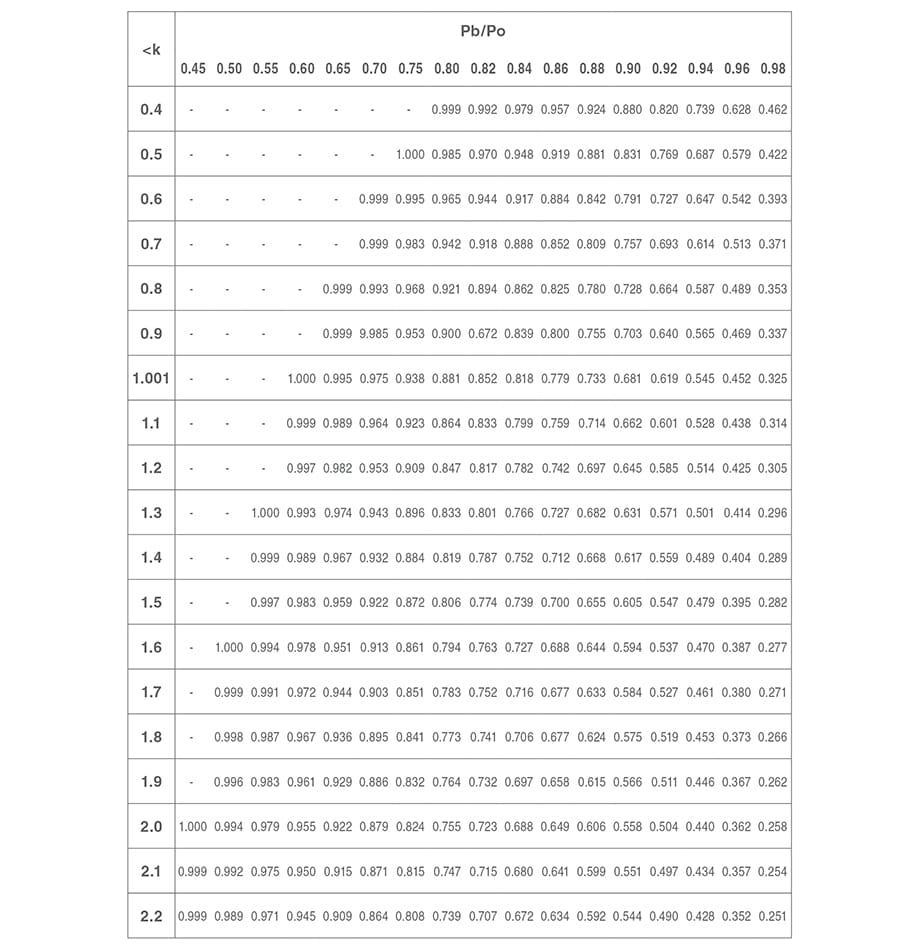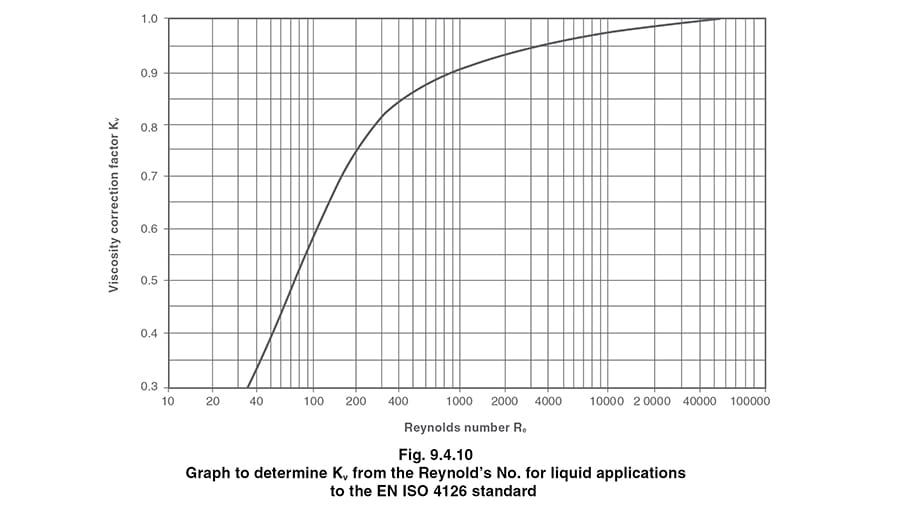Example 9.4.4

Size the minimum flow area required for a safety valve designed to EN ISO 4126 to relieve a superheated steam system of overpressure.

##### Steam system conditions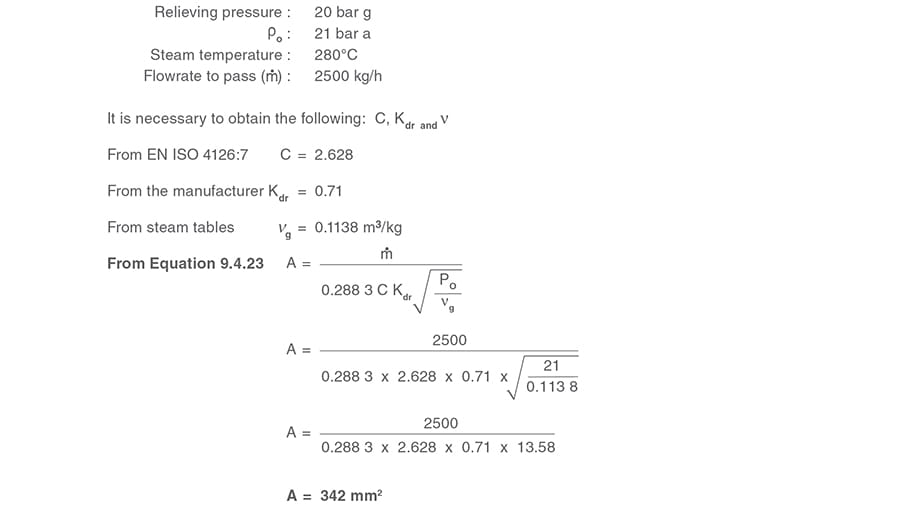##### Appendix A - Properties of industrial liquids

Table 9.4.5 Properties of some common industrial liquids

For specific gravity (G) used in ASME liquid sizing calculations, divide density by 998 (density of water).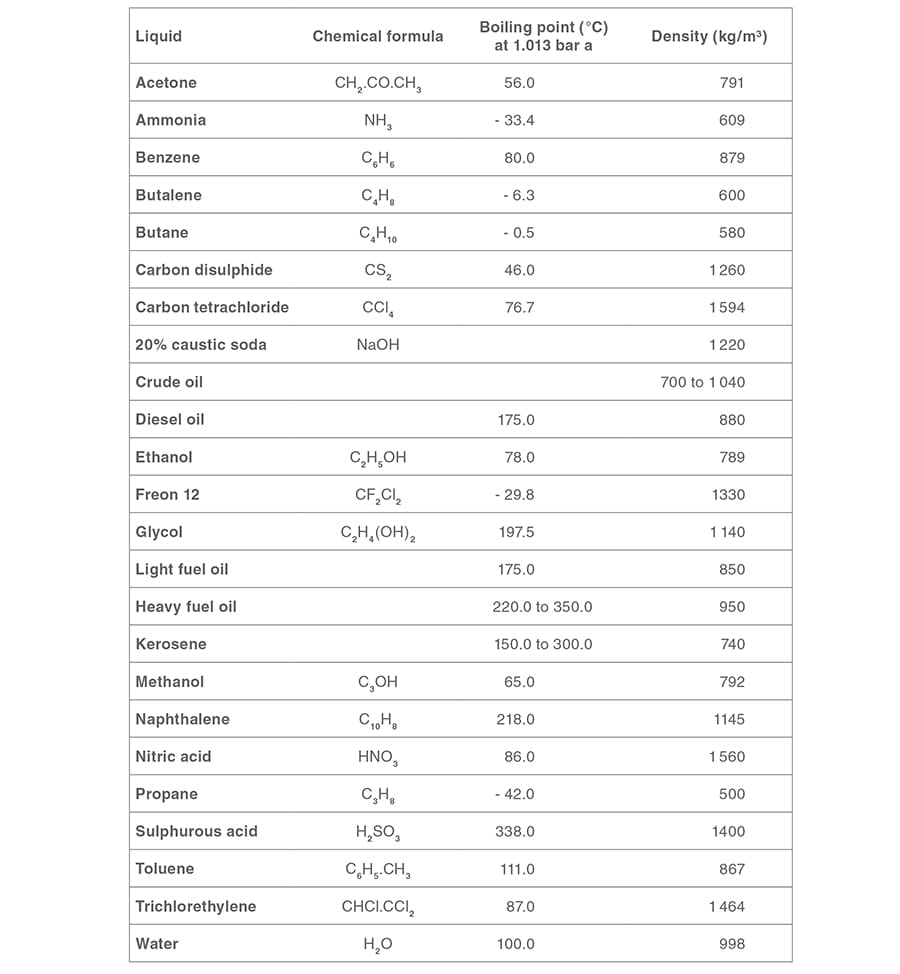##### Properties of industrial gases

Table 9.4.6 Properties of some common industrial gases

For specific gravity (G) used in ASME gas sizing calculations, divide molar mass by 28.96 (molar mass of air).